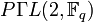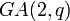# Difference between revisions of "Conjugacy class size formulas for linear groups of degree two"

This article gives formulas for the number of conjugacy classes as well as the sizes of individual conjugacy classes for the general linear group of degree two and some other related groups, both for a finite field of size$q$ and for some related rings.

## For a finite field of size$q$

In the formulas below, the field size is$q$. The characteristic of the field is a prime number$p$.$q$ is a prime power with underlying prime$p$. We let$r = \log_pq$, so$q = p^r$ and$r$ is a nonnegative integer.

### Formulas for number of conjugacy classes

Group Symbolic notation Formula for number of conjugacy classes in case of characteristic two (i.e.,$p = 2$)
Examples are$q = 2,4,8,16,\dots$
Formula for number of conjugacy classes in case of odd characteristic (equivalent to$q$ being odd).
Example are$q = 3,5,7,9,11,13,17,\dots$
Degree of polynomial in$q$ More information
general linear group of degree two$GL(2,q)$ or$GL(2,\mathbb{F}_q)$$q^2 - 1$$q^2 - 1$ 2 element structure of general linear group of degree two over a finite field
projective general linear group of degree two$PGL(2,q)$ or$PGL(2,\mathbb{F}_q)$$q + 1$$q + 2$ 1 element structure of projective general linear group of degree two over a finite field
special linear group of degree two$SL(2,q)$ or$SL(2,\mathbb{F}_q)$$q + 1$$q + 4$ 1 element structure of special linear group of degree two over a finite field
projective special linear group of degree two$PSL(2,q)$ or$PSL(2,\mathbb{F}_q)$$q + 1$$(q + 5)/2$ 1 element structure of projective special linear group of degree two over a finite field
general semilinear group of degree two$\Gamma L(2,q)$ or$\Gamma L(2,\mathbb{F}_q)$  ?  ? 2 (essentially, if we treat$r$ as a constant) element structure of general semilinear group of degree two over a finite field
projective semilinear group of degree two$P\Gamma L(2,q)$ or$P\Gamma L(2,\mathbb{F}_q)$  ?  ? 2 element structure of projective semilinear group of degree two over a finite field
general affine group of degree two$GA(2,q)$ or$AGL(2,q)$ or$GA(2,\mathbb{F}_q)$ or$AGL(2,\mathbb{F}_q)$$q^2 + q - 1$$q^2 + q - 1$ 2 element structure of general affine group of degree two over a finite field
special affine group of degree two$SA(2,q)$ or$ASL(2,q)$ or$SA(2,\mathbb{F}_q)$ or$ASL(2,\mathbb{F}_q)$  ?$2q + 4$ 1 element structure of special affine group of degree two over a finite field